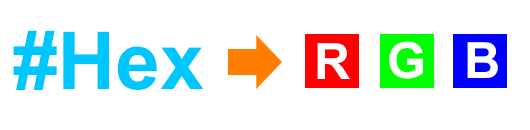# Hex to RGB(a) ConvertorInformation

## What is Hex to RGB(a)?

A hex triplet is a six-digit, three-byte hexadecimal number used in HTML, CSS, SVG, and other computing applications to represent colors. The bytes represent the red, green and blue components of the color. One byte represents a number in the range 00 to FF (in hexadecimal notation), or 0 to 255 in decimal notation. This represents the least (0) to the most (255) intensity of each of the color components. Thus web colors specify colors in the True Color (24-bit RGB) color scheme. The hex triplet is formed by concatenating three bytes in hexadecimal notation, in the following order:

Byte 1: red value (color type red)
Byte 2: green value (color type green)
Byte 3: blue value (color type blue)

For example:

A color in blue, i.e. #0000FF
Red = 0
Green = 0
Blue = 255

Note that if any one of the three color values is less than 16 (hex) or 10 (decimal), it must be represented with a leading zero so that the triplet always has exactly six digits. For example, the decimal triplet 4, 8, 16 would be represented by the hex digits 04, 08, 10, forming the hex triplet 040810.
The number of colors that can be represented by this system is 2563 or 224 or 166 = 16,777,216.

RGBa is a way to declare a color in CSS that includes alpha transparency support.